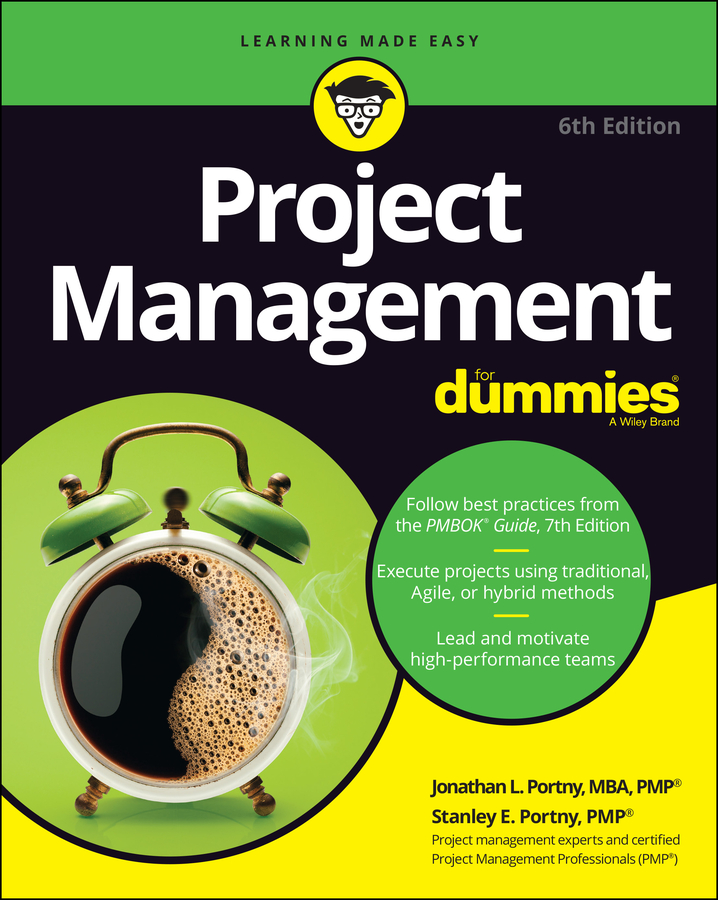##### Project Management For DummiesIf your project is fairly complex, you may consider using earned-value management (EVM) to help control performance. By providing cost and schedule performance assessments of both the total project and its major parts, EVM allows you to identify the likely problem areas so you can take the most effective corrective actions.

The following example presents a realistic illustration of how EVM can support insightful analysis of your project’s performance.

Suppose the Acme Company has awarded a contract for the production of two specialized and complex corporate brochures to Copies ‘R’ Us. The contract calls for Copies ‘R’ Us to produce 500 copies of Brochure A and 1,000 copies of Brochure B. It further states that Copies ‘R’ Us will produce Brochure A at the rate of 100 per month and Brochure B at the rate of 250 per month. Production of Brochure A is to start on January 1, and production of Brochure B is to start on February 1.

Here's the project plan:

Plan for Copies ‘R’ Us to Produce Brochures A and B
Activity Start End Elapsed Time Number of Copies Total Cost
Brochure A Jan 1 May 31 5 months 500 \$100,000
Brochure B Feb 1 May 31 4 months 1,000 \$100,000
Total \$200,000

A quick glance reveals that you budgeted \$200 a copy for Brochure A (\$100,000 / 500 copies) and \$100 a copy for Brochure B (\$100,000 / 1,000 copies).

Suppose it’s the end of March, and you’re three months into the project. Here’s what has happened as of March 31:

Project Status as of March 31
Activity Start Elapsed Time Number of Copies Produced Total Cost
Brochure A Jan 1 3 months 150 \$45,000
Brochure B Feb 1 2 months 600 \$30,000
Total \$75,000

Your job is to figure out your schedule and cost performances to date and to update your forecast of the total amount you’ll spend for both brochures. Follow these steps:

1. Determine the planned value (PV), earned value (EV), and actual cost (AC) for Brochure A through March 31 as follows:

PV = \$200 per brochure × 100 brochures per month × 3 months = \$60,000

EV = \$200 per brochure × 150 brochures = \$30,000

AC = \$45,000

2. Determine the schedule variance (SV), cost variance (CV), schedule performance index (SPI), and cost performance index (CPI) for the production of Brochure A through March 31 as follows:

SV = EV – PV = \$30,000 – \$60,000 = –\$30,000

CV = EV – AC = \$30,000 – \$45,000 = –\$15,000

SPI = EV / PV = \$30,000 / \$60,000 = 0.50

CPI = EV / AC = \$30,000 / \$45,000 = 0.67

Your analysis reveals that you produced only half of the copies of Brochure A you thought you would and that each brochure cost you 1.5 times the amount you had planned to spend (1 / CPI = 1 / 0.67 = 1.5; also, actual cost per brochure / planned cost per brochure = [\$45,000 expended / 150 brochures produced] / \$200 per brochure = 300 / 200 = 1.5).

3. Determine the planned value (PV), earned value (EV), and actual cost (AC) for Brochure B through March 31 as follows:

PV = \$100 per brochure × 250 brochures per month × 2 months = \$50,000

EV = \$100 per brochure ×× 600 brochures = \$60,000

AC = \$30,000

4. Determine the schedule variance (SV), cost variance (CV), schedule performance index (SPI), and cost performance index (CPI) for the production of Brochure B through March 31 as follows:

SV = EV – PV = \$60,000 – \$50,000 = \$10,000

CV = EV – AC = \$60,000 – \$30,000 = \$30,000

SPI = EV / PV = \$60,000 / \$50,000 = 1.20

CPI = EV /AC = \$60,000 / \$30,000 = 2.00

Your analysis reveals that production of Brochure B is 20 percent ahead of schedule and 50 percent under budget (1 / CPI = 1 / 2 = 0.5; also, [\$30,000 expended / 600 brochures produced] / \$100 per brochure = 50 / 100 = 0.5).

5. Forecast the estimate at completion (EAC) for Brochure A.

Assume the remaining work is performed at the originally budgeted rate.

EAC = BAC + AC – EV = \$100,000 + \$45,000 – \$30,000 = \$115,000

6. Forecast the estimate at completion (EAC) for Brochure B.

Assume the remaining work is performed at the originally budgeted rate.

EAC = BAC + AC – EV = \$100,000 + \$30,000 – \$60,000 = \$70,000

7. Determine the overall status of your project by adding together the schedule variances (SV), the cost variances (CV), and the updated estimates at completion (EAC) for Brochures A and B.

Project SV = –\$30,000 + \$10,000 = –\$20,000

Project CV = –\$15,000 + \$30,000 = \$15,000

Assume the remaining work is performed at the originally budgeted rate.

EAC = \$115,000 + \$70,000 = \$185,000

If project production rates and costs remain the same until all the required brochures are produced you’ll finish on budget five months late.